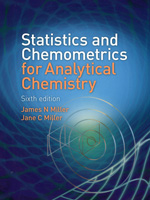Statistics & Chemometrics for Analytical Chemistry,
6th edition

By James N. Miller & Jane C. Miller
August 2010
Pearson Education
Distributed by Trans-Atlantic Publications
ISBN: 9780273730422
278 pages, Illustrated
\$97.50 Paper Original1. Introduction
1.1 Analytical problems
1.2 Errors in qunatitative analysis
1.3 Types of error
1.4 Random and systematic errors in titrimetric analysis
1.5 Handling systematic errors
1.6 Planning and design of experiments
1.7 Calculators and computers in statistical calculations
2. Statistics of Repeated Measurements
2.1 Mean and standard deviation
2.2 The distribution of repeated measurements
2.3 Log-normal distribution
2.4 Definition of a 'sample'
2.5 The sampling distribution of the mean

2.6 Confidence limits of the mean for large samples
2.7 Confidence limits of the mean for small samples
2.8 Presentation of results
2.9 Other uses of confidence limits
2.10 Confidence limits of the geometric mean for a log-normal distribution
2.11 Propagation of random errors
2.12 Propagation of systematic errors
3. Significance Tests
3.1 Introduction
3.2 Comparison of an experimental mean with a known value
3.3 Comparison of two experimental means
3.4 Paired t-test
3.5 One-sided and two-sided tests
3.6 F-test for the comparison of standard deviations
3.7 Outliers
3.8 Analysis of variance
3.9 Comparison of several means
3.10 The arithmetic of ANOVA calculations
3.11 The chi-squared test
3.12 Testing for normality of distribution
3.13 Conclusions from significance tests

3.14 Bayesian Statistics
4. The Quality of Analytical Measurements
4.1 Introduction
4.2 Sampling
4.3 Separation and estimation of variances using ANOVA
4.4 Sampling strategy
4.5 Quality control methods - Introduction
4.6 Stewhart charts for mean values
4.7 Stewhart charts for ranges
4.8 Establishing the process capability
4.9 Average run length: cusum charts
4.10 Zone control charts (J-charts)

4.11 Proficiency testing schemes
4.12 Method performance studies (collaborative trials)
4.13 Uncertainty
4.14 Acceptable sampling

4.15 Method validation
5. Calibration Methods in Instumental Analysis
5.1 Introduction: instrumentational analysis
5.2 Calibration graphs in instrumental analysis
5.3 The product-moment correlation coefficient
5.4 The line of regression of y on x
5.5 Errors in the slope and intercept of the regression line
5.6 Calculation of a concentration and its random error
5.7 Limits of detection
5.8 The method of standard additions
5.9 Use of regression lines for comparing analytical methods
5.10 Weighted regression lines
5.11 Intersection of two straight lines
5.12 ANOVA and regression calculations
5.13 Curvilinear regression methods - Introduction
5.14 Curve fitting
5.15 Outliers in regression
6. Non-parametric and Robust Methods
6.1 Introduction
6.2 The median: initial data analysis
6.3 The sign test
6.4 The Wald-Wolfowitz runs test
6.5 The Wilcoxon signed rank test
6.6 Simple tests for two independent samples
6.7 Non-parametric tests for more than two samples
6.8 Rank correlation
6.9 Non-parametric regression methods
6.10 Robust methods: introduction
6.11 Simple robust methods: trimming and winsorization

6.12 Further robust estimates of location and spread

6.13 Robust ANOVA

6.14 Robust regression methods

6.15 Re-sampling statistics

6.16 Conclusions
7. Experiimental Design and Optimization
7.1 Introduction
7.2 Randomization and blocking
7.3 Two-way ANOVA
7.4 Latin squares and other designs
7.5 Interactions
7.6 Identifying the important factors: factorial designs

7.7 Fractional factorial designs
7.8 Optimization: basic principles and univariate methods
7.9 Optimization using the alternating variable search method
7.10 The method of steepest ascent
7.11 Simplex optimization
7.12 Simulated annealing
8. Multivariate Analysis
8.1 Introduction
8.2 Initial analysis
8.3 Prinicipal component analysis
8.4 Cluster analysis
8.5 Discriminant analysis
8.6 K-nearest neighbour method
8.7 Disjoint class modelling
8.8 Regression methods

8.9 Multiple linear regression
8.10 Principal component regression
8.11 Partial least squares regression

8.12 Natural computation methods; artificial neural networks

8.13 Conclusions
Solutions to Exercises
Appendix 1 Commonly used statistical significance tests
Appendix 2 Statistical tables
Index